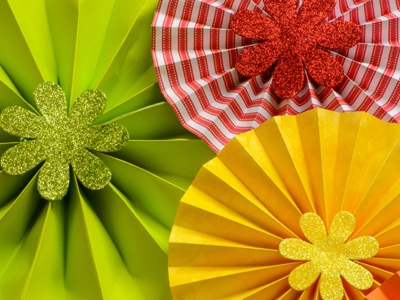The circumference of a circle is the distance all round the edge of it.

# Words Used in Maths 01

Perimeter and prime number are words predominantly used in Maths. Like other subjects, or fields of knowledge, Maths uses various English words in a specialised manner that you need to know in order to learn the subject.

Many of these words hold meanings similar to general usage, although you wouldn't want to confuse the maths terminology with the every day understanding of a word. One example is "face". The most common meaning of the term is that part of the body where the eyes, nose and mouth are located, but in maths, of course, a "face" is a flat surface of a 3D object. You can see how the two definitions are related, but it is important to bear the differences in mind, too.

How much mathematical vocabulary do you know? Geometrical terms include angle, line, plane, axis, circumference, radius, vertices, acute, obtuse, pi, parallel, perpendicular, etc. Vocabulary necessary for calculations includes numerator, denominator, fraction, decimal, quotient, product, square, square root, divisible, etc. Many mathematical terms come from other languages, such as Latin and Greek.

Have a go at this quiz to get you up to speed with mathematical terms and spelling.

1.
Choose the correct meaning for the following word(s).
Convex
Curving inwards
Curving left
Curving outwards
Curving right
"Convex" is the opposite of "concave"
2.
Choose the correct meaning for the following word(s).
Quotient
Result of adding one number to another
Result of dividing one number by another
Result of multiplying one number by another
Result of subtracting one number from another
The quotient of 4 divided by 2 is 2!
3.
Choose the correct meaning for the following word(s).
Perpendicular
At a reflex angle to a line
At a right angle to a line
At an acute angle to a line
At an obtuse angle to a line
Make sure you can spell "perpendicular" correctly
4.
Choose the correct meaning for the following word(s).
Circumference
Distance all round the edge of a circle
Distance halfway round the edge of a circle
Distance part of the way round the edge of a circle
Distance quarter of the way round the edge of a circle
The latin prefix circum- means "round"
5.
Choose the correct meaning for the following word(s).
Product
Result of adding two or more numbers
Result of dividing two or more numbers
Result of multiplying two or more numbers
Result of subtracting two or more numbers
The product of 2 x 2 is 4
6.
Choose the correct meaning for the following word(s).
Prime number
Number divisible only by itself and one
Number divisible only by itself and two
Number divisible only by itself and three
Number divisible only by itself and four
Some examples of prime numbers are 3, 5, 7, 11, 13
7.
Choose the correct meaning for the following word(s).
Integer
One letter
One whole number
The whole
The whole range of letters
"Integer" means "whole number" (not a fraction)
8.
Choose the correct meaning for the following word(s).
Concave
Curving inwards
Curving left
Curving outwards
Curving right
A cave curves inwards!
9.
Choose the correct meaning for the following word(s).
Diameter
Straight line a part of the way across a circle
Straight line a quarter of the way across a circle
Straight line halfway across the middle of a circle
Straight line right across the middle of a circle
Don't confuse "diameter" with "radius" (half diameter)
10.
Choose the correct meaning for the following word(s).
Perimeter
All round the edge of a shape
Halfway round the edge of a shape
Part of the way round the edge of a shape
Quarter of the way round the edge of a shape
The greek prefix peri- means "round"

Author:  Sue Daish